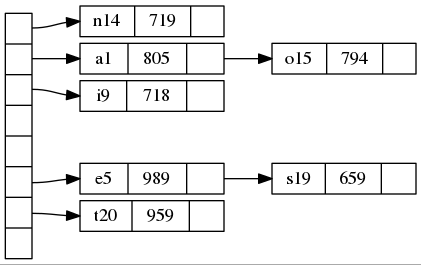[TOC]

# dot 绘图语言

graphviz 是一个很古老的开源软件包了，用来绘制结构化的图形。

# 1. 有向图和无向图

``````digraph G{
a -> b;
}``````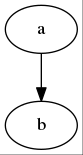``````graph G{
a -- b;
}``````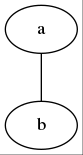# 2. 边和节点

``````digraph G{
a;
b;
}``````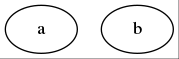``````digraph G{
a -> b;
}````````````digraph G{
a -> {b c};
}``````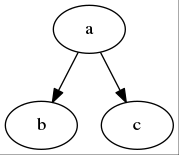``````digraph G{
{a b c} -> {d e f};
}``````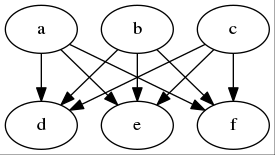``````digraph G{
a -> b -> {c d};
}``````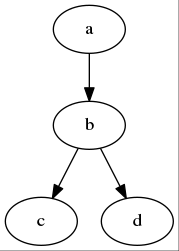# 3. 设置属性

## 3.1. 对单个节点或边设置属性

``````digraph G{
a [label="节点 A"];
b [label="节点 B"];
a -> b [label="从 A 到 B"];
}``````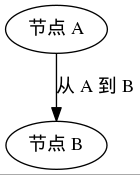``````digraph G{
a [label="节点 A", shape=circle];
b [label="节点 B", shape=doublecircle];
a -> b [label="从 A 到 B", arrowhead=vee];
}``````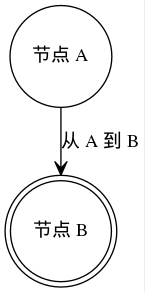## 3.2. 对所有的节点或边设置属性

``````digraph G{
node[shape=circle];
a [label="节点 A"];
b [label="节点 B"];
a -> b [label="从 A 到 B"];
}``````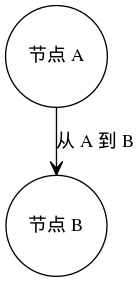## 3.3. 设置图的属性

``````digraph G{
rankdir = LR;
label = "示例图";
a -> b;
}``````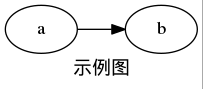rankdir 设置了图的布局为 LR，即从左向右；默认图的布局是 TB，即从上向下。 label 设置了对图本身的说明标签。

# 4. 注释

dot 支持 C 和 C++风格的注释，//和/* */两种。

# 5. 复杂的节点结构

``````digraph structs {
node [shape=record];
struct1 [shape=record,label="<f0> left|<f1> mid\ dle|<f2> right"];
struct2 [shape=record,label="<f0> one|<f1> two"];
struct3 [shape=record,label="hello\nworld |{ b |{c|<here> d|e}| f}| g | h"];
struct1 -> struct2;
struct1 -> struct3;
}``````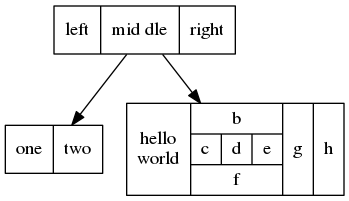``````digraph structs {
node [shape=record];
struct1 [shape=record,label="<f0> left|<f1> middle|<f2> right"];
struct2 [shape=record,label="<f0> one|<f1> two"];
struct3 [shape=record,label="hello\nworld |{ b |{c|<here> d|e}| f}| g | h"];
struct1:f1 -> struct2:f0;
struct1:f2 -> struct3:here;
}``````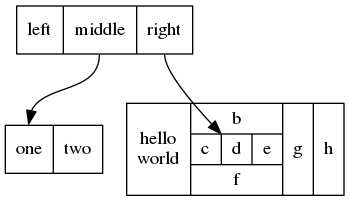# 6. 子图

``````digraph graphname
{
a -> {b c};
c -> b;
subgraph cluster_bc
{
bgcolor=red;
label="cluster";
b;
c;
}
}``````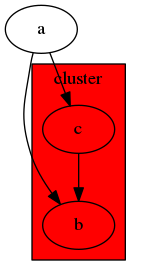# 7. 几个示例

## 7.1. 函数调用图

``````digraph G {
size ="4,4";
main [shape=box]; /* this is a comment */
main -> parse [weight=8];
parse -> execute;
main -> init [style=dotted];
main -> cleanup;
execute -> { make_string; printf}
init -> make_string;
edge [color=red]; // so is this
main -> printf [style=bold,label="100 times"];
make_string [label="make a\nstring"];
node [shape=box,style=filled,color=".7 .3 1.0"];
execute -> compare;
}``````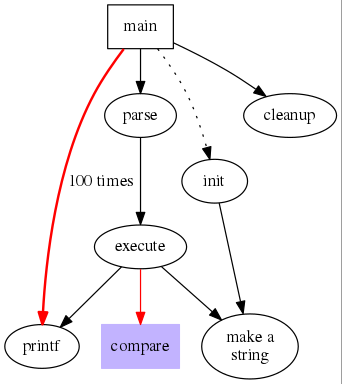## 7.2. 数据结构二叉树

``````digraph g {
node [shape = record,height=.1];
node0[label ="<f0> |<f1> G|<f2>"];
node1[label ="<f0> |<f1> E|<f2>"];
node2[label ="<f0> |<f1> B|<f2>"];
node3[label ="<f0> |<f1> F|<f2>"];
node4[label ="<f0> |<f1> R|<f2>"];
node5[label ="<f0> |<f1> H|<f2>"];
node6[label ="<f0> |<f1> Y|<f2>"];
node7[label ="<f0> |<f1> A|<f2>"];
node8[label ="<f0> |<f1> C|<f2>"];
"node0":f2 ->"node4":f1;
"node0":f0 ->"node1":f1;
"node1":f0 ->"node2":f1;
"node1":f2 ->"node3":f1;
"node2":f2 ->"node8":f1;
"node2":f0 ->"node7":f1;
"node4":f2 ->"node6":f1;
"node4":f0 ->"node5":f1;
}``````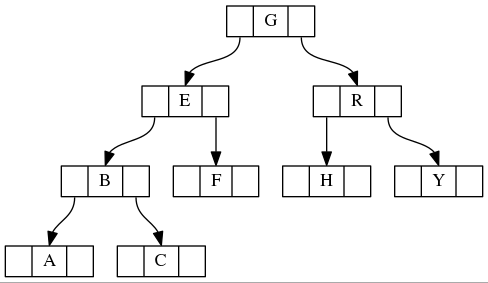## 7.3. 数据结构 hash 表

``````digraph G {
nodesep=.05;
rankdir=LR;
node [shape=record,width=.1,height=.1];

node0 [label = "<f0> |<f1> |<f2> |<f3> |<f4> |<f5> |<f6> | ",height=2.5];
node [width = 1.5];
node1 [label = "{<n> n14 | 719 |<p> }"];
node2 [label = "{<n> a1  | 805 |<p> }"];
node3 [label = "{<n> i9  | 718 |<p> }"];
node4 [label = "{<n> e5  | 989 |<p> }"];
node5 [label = "{<n> t20 | 959 |<p> }"] ;
node6 [label = "{<n> o15 | 794 |<p> }"] ;
node7 [label = "{<n> s19 | 659 |<p> }"] ;

node0:f0 -> node1:n;
node0:f1 -> node2:n;
node0:f2 -> node3:n;
node0:f5 -> node4:n;
node0:f6 -> node5:n;
node2:p -> node6:n;
node4:p -> node7:n;
}``````